# PivotGridCustomTotalBase.SummaryType Property

Gets or sets the type of the summary function used to calculate the current custom total.

Namespace: DevExpress.XtraPivotGrid

Assembly: DevExpress.PivotGrid.v22.2.Core.dll

NuGet Packages: DevExpress.PivotGrid.Core, DevExpress.Win.Dashboard.Design

## Declaration

``````[DefaultValue(PivotSummaryType.Sum)]
public PivotSummaryType SummaryType { get; set; }``````

## Property Value

Type Default Description
PivotSummaryType Sum

A PivotSummaryType enumeration value that specifies the summary function type.

Available values:

Show 13 items
Name Description
Count

The number of values (excluding Null and DBNull values).

Sum

The sum of the values.Min

The smallest value.

Max

The largest value.

Average

The average of the values.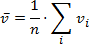StdDev

An estimate of the standard deviation of a population, where the sample is a subset of the entire population.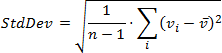StdDevp

The standard deviation of a population, where the population is all of the data to be summarized.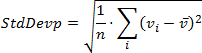Var

An estimate of the variance of a population, where the sample is a subset of the entire population.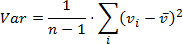Varp

The variance of a population, where the population is all of the data to be summarized.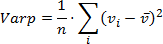Custom

Specifies whether calculations should be performed manually, by handling the CustomSummary event.

Not supported in OLAP and server mode.

CountDistinct

The number of distinct values. This function works only in the Optimized mode.

Median

The median of the values. A median is the number separating the higher half of a value range from the lower half. This function works only in the Optimized mode.

Mode

The mode of the values. A mode is the value that is repeated more often than any other. This function works only in the Optimized mode.

## Remarks

The XtraPivotGrid control calculates custom totals against data fields. The type of a custom total’s summary function is determined by the SummaryType property.

For data fields which don’t contain numeric data only the following summary functions can be calculated: PivotSummaryType.Count, PivotSummaryType.Min and PivotSummaryType.Max. If the field’s SummaryType property is set to any other value the corresponding data cells will display zeros.

For numeric data fields any summary function type listed by the PivotSummaryType enumerator can be calculated.

Note

The PivotSummaryType.Custom summary type is not supported in OLAP mode.